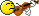# Number argument to linux command from root script

Hello,

I want to give a user defined number as an argument to the Linux command in a root script.
Could you please let me know how it can be done?

Here is an example:

Int_t K = 12107;
gSystem->Exec(“kill -9 K”); //where “K” is process id.

I know above statement won’t work.

Ajay

VIVE L’AMOUR!
try to play with TString: http://root.cern.ch/root/htmldoc/TString.htmlroot  TString command = "kill -9 "; // note the space in the end of the string
root  cout << command << endl;
kill -9
root  cout << (TString(command) += 12107) << endl; // we create a copy of the “command”
kill -9 12107
root  Int_t K = 12107;
root  cout << (TString(command) += K).Data() << endl; // we create a copy of the “command”
kill -9 12107
root  gSystem->Exec((TString(command) += K).Data()); //where “K” is process id.
sh: line 0: kill: (12107) - No such process
root  cout << command << endl; // note that we did not modify the original “command” string
kill -9

In fact you don’t even need to create the “command”. You can simply write:root  Int_t K = 12107;
root  gSystem->Exec((TString("kill -9 ") += K).Data()); //where “K” is process id.
sh: line 0: kill: (12107) - No such process

I am stupid. No?
Pepe Le Pew.

``gSystem->Exec ( Form("kill -9 %d", K ) );``

Is the easiest (although I believe not thread-safe…).

Cheers,
Charles

`.Data()` is not needed.

I know “.Data()” is usually not needed (and it is not needed in my examples in this thread), but I have met cases where it was necessary (for no obvious reason). So, I always explicitly add it to what I write as examples. Sooner or later one will get a fairly cryptic error, and adding “.Data()” will be the solution.

It’s worth making sure you look to see if a given function can take a TString as an argument or needs a const char*. If you don’t need to pass a const char* and can use a more civilized type (e.g., std::string or TString), then that’s what one should use.

Cheers,
Charles

That’s more or less what I am trying to follow. In case the function/method expects a “const char *” parameter I always add “.Data()” (or “->Data()”) in my examples (and “TSystem::Exec” is such a method), even if I know that it’s not really needed (as “TSystem::Exec” will also happily eat a TString parameter). For unknown reasons, I have had cases in which the “automatic mechanism of type conversion” (from TString to “const char *”) did not work and I needed to manually add “.Data()” (or “->Data()”). As far as I remember this always happened in compiled code (i.e. the compiler issued errors).

Hi All,

Thanks a lot!
That is what I was looking for. It really helped.

Ajay